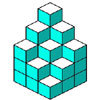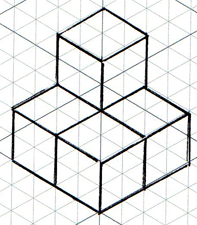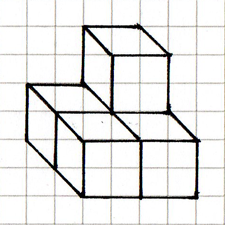#### You may also like### Soma - So Good

Can you mentally fit the 7 SOMA pieces together to make a cube? Can you do it in more than one way?### Nine Colours

Can you use small coloured cubes to make a 3 by 3 by 3 cube so that each face of the bigger cube contains one of each colour?### Take Ten

Is it possible to remove ten unit cubes from a 3 by 3 by 3 cube so that the surface area of the remaining solid is the same as the surface area of the original?

# Isometric Drawing

##### Age 11 to 14Challenge LevelDrawing in isometric perspective preserves:
• relative proportions of lengths of edges
• parallel lines
It does not preserve:
• angles between edges - they are actually all right angles, but the isometric grid means all angles are multiples of 60 degrees
• perpendicular lines, because there are no right angles on an isometric grid

Comparing this isometric representation of the structure with the same structure represented in oblique projection (below left):
•relative proportions of lengths of edges are preserved in the isometric drawing but not in oblique perspective
• neither preserves angles
• both preserve parallel lines
• neither preserve perpendicular lines

One advantage of isometric perspective is that relative proportions of lengths are preserved - if lengths are drawn equal, or if one length is drawn twice as long as another, then that is also the case in reality.
A disadvantage is that there is no way of showing a structure from a slightly oblique direction - the drawing above suggests that the structure is positioned symmetrically relative to the observer, whereas the original photo shows the right hand faces facing toward the observer, and the left hand faces facing away from the observer.

One advantage of oblique perspective is the relative ease of drawing a structure using this method.
A disadvantage is that no information from the original structure is preserved.